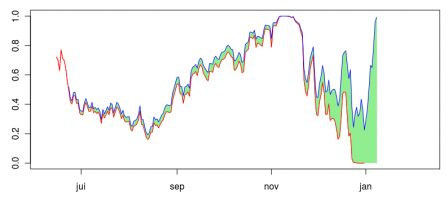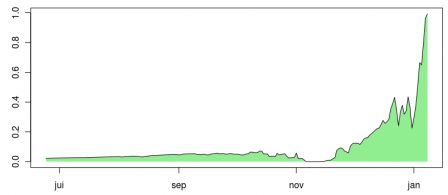# From one extreme (0) to another (1): challenge failed, but who cares…

January 9, 2011
By

Want to share your content on R-bloggers? click here if you have a blog, or here if you don't.

Just after arriving in Montréal, at the beginning of September, I
discussed statistics of my blog, and said that it might be possible – or
likely – that by new year’s Eve, over a million page would have been
viewed on my blog (from Google’s counter, here). By the end of October (here) I was very optimistic, but mi-December (here) the challenge was likely to be failed. An indeed, the million page target was hit one week after, on January 8th,

`base=read.table("http://freakonometrics.blog.free.fr/public/data/million1.csv",sep="t",header=TRUE)X1=cumsum(base\$nombre)X0=X1base=read.table("http://freakonometrics.blog.free.fr/public/data/million2.csv",sep="t",header=TRUE)X2=cumsum(base\$nombre)X=X1+X2 D0=as.Date("08/11/2008","%d/%m/%Y")D=D0+1:length(X1)plot(D,X1,xlim=c(as.Date("08/06/2010","%d/%m/%Y"),as.Date("08/02/2011","%d/%m/%Y")),ylim=c(800000,1050000))abline(h=1000000,col="red")abline(v=as.Date("01/01/2011","%d/%m/%Y"),col="red")points(D,X,col="blue")`Again, the black points were from the previous blog (http://blogperso.univ-rennes1.fr/arthur.charpentier/) which was transferred to that new one (http://freakonometrics.blog.free.fr) this Autumn. So I just sum up the stats to get the blue points.
At each date, I fit an ARIMA, and use it to make forecast the
total number of pages viewed on January 1st, and calculate the
probability to reach a million page viewed at that date (using a
Gaussian ARIMA model). Actually, here, I changed a little bit the
challenge, and asked “what would have been the probability to reach a million page viewed on January 1st, and on January 8th” ?

`kt=which(D==as.Date("01/06/2010","%d/%m/%Y"))Xbase=XX=X1+X2P1=P2=rep(NA,(length(X)-kt)+7)for(h in 0:(length(X)-kt+7)){model  <- arima(X[1:(kt+h)],c(7 ,1,7),method="CSS")forecast <- predict(model,200) u=max(D[1:kt+h])+1:300if(min(u)<=as.Date("01/01/2011","%d/%m/%Y")){k=which(u==as.Date("01/01/2011","%d/%m/%Y"))(P1[h+1]=1-pnorm(1000000,forecast\$pred[k],forecast\$se[k]))}k=which(u==as.Date("08/01/2011","%d/%m/%Y"))(P2[h+1]=1-pnorm(1000000,forecast\$pred[k],forecast\$se[k]))}`

The red curve is the
probability to reach 1 million viewed on January 1st (as done earlier,
using an ARIMA projection). The blue one is
the probability to reach 1 million viewed one week after, on January 8th.and here is the difference between probabilities,The flat part at the beginning of November corresponds to the bump that
was observed on the initial graph. But then, the slope was too low, and
in December, the challenge was failed… Obviously, looking at statistics during a blog migration is not a bright idea…

R-bloggers.com offers daily e-mail updates about R news and tutorials about learning R and many other topics. Click here if you're looking to post or find an R/data-science job.
Want to share your content on R-bloggers? click here if you have a blog, or here if you don't.

Tags: , , , , , , , , ,